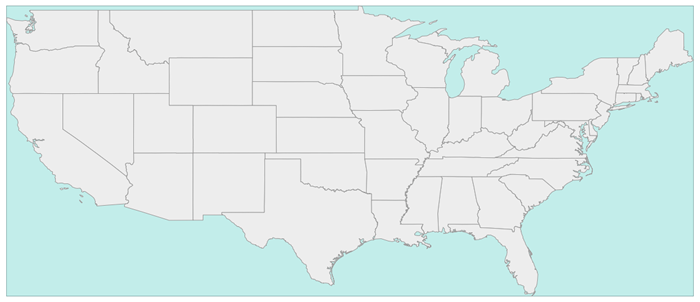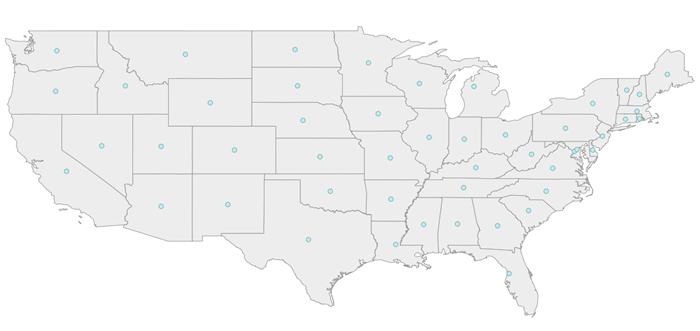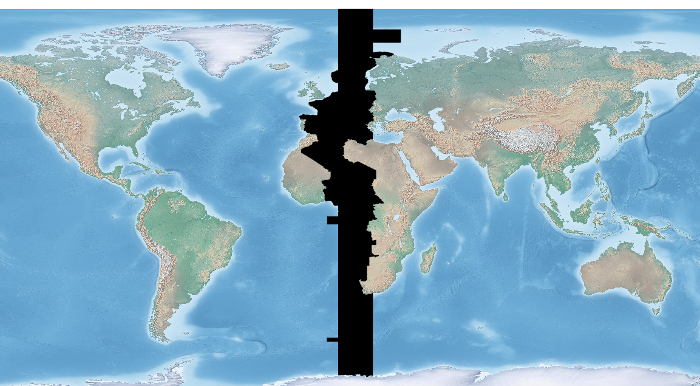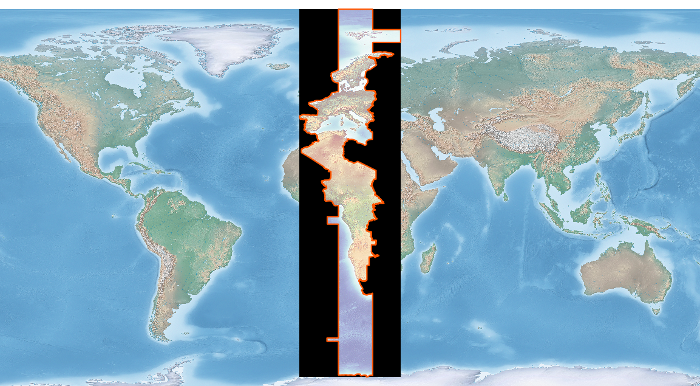# Layers¶

## Is Ogr Installed¶

```try:
from osgeo import ogr
print 'Import of ogr from osgeo worked.  Hurray!\n'
except:
print 'Import of ogr from osgeo failed\n\n'
```

## View Auto Generated Ogr Help¶

This code simply prints out the auto-generated help on the imported module. In this case it’s OGR.
```import osgeo.ogr
print help(osgeo.ogr)
```

## Get List of Ogr Drivers Alphabetically (A- Z)¶

It’s always driven me a little nuts that the command line ogr2ogr –formats returns a ‘random’ list of drivers. This code returns the list of OGR drivers alphabetically from A - Z. .
```import ogr
cnt = ogr.GetDriverCount()
formatsList = []  # Empty List

for i in range(cnt):
driver = ogr.GetDriver(i)
driverName = driver.GetName()
if not driverName in formatsList:
formatsList.append(driverName)

formatsList.sort() # Sorting the messy list of ogr drivers

for i in formatsList:
print i
```

## Is Ogr Driver Available by Driver Name¶

This code shows if a particular OGR driver is available. The exact names are the ones used on the OGR Vector Formats page in the “Code” column ([formats website]). This is the same names returned when you enter ogrinfo --formats on the command line.

Code Example Source: [website]

```from osgeo import ogr

## Shapefile available?
driverName = "ESRI Shapefile"
drv = ogr.GetDriverByName( driverName )
if drv is None:
print "%s driver not available.\n" % driverName
else:
print  "%s driver IS available.\n" % driverName

## PostgreSQL available?
driverName = "PostgreSQL"
drv = ogr.GetDriverByName( driverName )
if drv is None:
print "%s driver not available.\n" % driverName
else:
print  "%s driver IS available.\n" % driverName

## Is File GeoDatabase available?
driverName = "FileGDB"
drv = ogr.GetDriverByName( driverName )
if drv is None:
print "%s driver not available.\n" % driverName
else:
print  "%s driver IS available.\n" % driverName

## SDE available?
driverName = "SDE"
drv = ogr.GetDriverByName( driverName )
if drv is None:
print "%s driver not available.\n" % driverName
else:
print  "%s driver IS available.\n" % driverName
```

## Get Shapefile Feature Count¶

This code example opens a shapefile and returns the number of features in it. Solution mined from: [web site]
```import os
from osgeo import ogr

daShapefile = r"C:\Temp\Voting_Centers_and_Ballot_Sites.shp"

driver = ogr.GetDriverByName('ESRI Shapefile')

dataSource = driver.Open(daShapefile, 0) # 0 means read-only. 1 means writeable.

# Check to see if shapefile is found.
if dataSource is None:
print 'Could not open %s' % (daShapefile)
else:
print 'Opened %s' % (daShapefile)
layer = dataSource.GetLayer()
featureCount = layer.GetFeatureCount()
print "Number of features in %s: %d" % (os.path.basename(daShapefile),featureCount)
```

## Get All PostGIS layers in a PostgreSQL Database¶

This returns all the layers in a database of your choosing sorted in alphabetical order (of course). Just fill in the missing information and it should work.
```from osgeo import ogr

databaseServer = "<IP of database server OR Name of database server"
databaseName = "<Name of database>"
databaseUser = "<User name>"

connString = "PG: host=%s dbname=%s user=%s password=%s" %(databaseServer,databaseName,databaseUser,databasePW)

conn = ogr.Open(connString)

layerList = []
for i in conn:
daLayer = i.GetName()
if not daLayer in layerList:
layerList.append(daLayer)

layerList.sort()

for j in layerList:
print j

conn.Destroy()
```

## Get PostGIS Layer Feature Count By Layer Name¶

This code example opens a postgis connection and gets the specified layer name if it exists in the database. Otherwise it throws a nice error message
```from osgeo import ogr
import sys

databaseServer = "<IP of database server OR Name of database server"
databaseName = "<Name of database>"
databaseUser = "<User name>"
connString = "PG: host=%s dbname=%s user=%s password=%s" % (databaseServer,databaseName,databaseUser,databasePW)

def GetPGLayer( lyr_name ):
conn = ogr.Open(connString)

lyr = conn.GetLayer( lyr_name )
if lyr is None:
print >> sys.stderr, '[ ERROR ]: layer name = "%s" could not be found in database "%s"' % ( lyr_name, databaseName )
sys.exit( 1 )

featureCount = lyr.GetFeatureCount()
print "Number of features in %s: %d" % ( lyr_name , featureCount )

conn.Destroy()

if __name__ == '__main__':

if len( sys.argv ) < 2:
print >> sys.stderr, '[ ERROR ]: you must pass at least one argument -- the layer name argument'
sys.exit( 1 )

lyr_name = sys.argv
GetPGLayer( lyr_name )
```

## Iterate over Features¶

```from osgeo import ogr
import os

shapefile = "states.shp"
driver = ogr.GetDriverByName("ESRI Shapefile")
dataSource = driver.Open(shapefile, 0)
layer = dataSource.GetLayer()

for feature in layer:
print feature.GetField("STATE_NAME")
```

## Get Geometry from each Feature in a Layer¶

```from osgeo import ogr
import os

shapefile = "states.shp"
driver = ogr.GetDriverByName("ESRI Shapefile")
dataSource = driver.Open(shapefile, 0)
layer = dataSource.GetLayer()

for feature in layer:
geom = feature.GetGeometryRef()
print geom.Centroid().ExportToWkt()
```

## Filter by attribute¶

```from osgeo import ogr
import os

shapefile = "states.shp"
driver = ogr.GetDriverByName("ESRI Shapefile")
dataSource = driver.Open(shapefile, 0)
layer = dataSource.GetLayer()

layer.SetAttributeFilter("SUB_REGION = 'Pacific'")

for feature in layer:
print feature.GetField("STATE_NAME")
```

## Spatial Filter¶

```from osgeo import ogr
import os

shapefile = "states.shp"
driver = ogr.GetDriverByName("ESRI Shapefile")
dataSource = driver.Open(shapefile, 0)
layer = dataSource.GetLayer()

wkt = "POLYGON ((-103.81402655265633 50.253951270672125,-102.94583419409656 51.535568561879401,-100.34125711841725 51.328856095555651,-100.34125711841725 51.328856095555651,-93.437060743203844 50.460663736995883,-93.767800689321859 46.450441890315041,-94.635993047881612 41.613370178339181,-100.75468205106476 41.365315218750681,-106.12920617548238 42.564247523428456,-105.96383620242338 47.277291755610058,-103.81402655265633 50.253951270672125))"
layer.SetSpatialFilter(ogr.CreateGeometryFromWkt(wkt))

for feature in layer:
print feature.GetField("STATE_NAME")
```

## Get Shapefile Fields - Get the user defined fields¶

This code example returns the field names of the user defined (created) fields.
```from osgeo import ogr

daShapefile = r"C:\Temp\Voting_Centers_and_Ballot_Sites.shp"

dataSource = ogr.Open(daShapefile)
daLayer = dataSource.GetLayer(0)
layerDefinition = daLayer.GetLayerDefn()

for i in range(layerDefinition.GetFieldCount()):
print layerDefinition.GetFieldDefn(i).GetName()
```

## Get Shapefile Fields and Types - Get the user defined fields¶

This code example returns the field names of the user defined (created) fields and the data types they are.
```from osgeo import ogr

daShapefile = r"C:\Temp\iDay\CWI_Wetlands.shp"

dataSource = ogr.Open(daShapefile)
daLayer = dataSource.GetLayer(0)
layerDefinition = daLayer.GetLayerDefn()

print "Name  -  Type  Width  Precision"
for i in range(layerDefinition.GetFieldCount()):
fieldName =  layerDefinition.GetFieldDefn(i).GetName()
fieldTypeCode = layerDefinition.GetFieldDefn(i).GetType()
fieldType = layerDefinition.GetFieldDefn(i).GetFieldTypeName(fieldTypeCode)
fieldWidth = layerDefinition.GetFieldDefn(i).GetWidth()
GetPrecision = layerDefinition.GetFieldDefn(i).GetPrecision()

print fieldName + " - " + fieldType+ " " + str(fieldWidth) + " " + str(GetPrecision)
```

## Get PostGIS Layer Fields - Get the user defined fields¶

This code example returns the field names of the user defined (created) fields.
```from osgeo import ogr
import sys

databaseServer = "<IP of database server OR Name of database server"
databaseName = "<Name of database>"
databaseUser = "<User name>"
connString = "PG: host=%s dbname=%s user=%s password=%s" %(databaseServer,databaseName,databaseUser,databasePW)

def GetPGLayerFields( lyr_name ):
conn = ogr.Open(connString)

lyr = conn.GetLayer( lyr_name )
if lyr is None:
print >> sys.stderr, '[ ERROR ]: layer name = "%s" could not be found in database "%s"' % ( lyr_name, databaseName )
sys.exit( 1 )

lyrDefn = lyr.GetLayerDefn()

for i in range( lyrDefn.GetFieldCount() ):
print lyrDefn.GetFieldDefn( i ).GetName()

conn.Destroy()

if __name__ == '__main__':

if len( sys.argv ) < 2:
print >> sys.stderr, '[ ERROR ]: you must pass at least one argument -- the layer name argument'
sys.exit( 1 )

lyr_name = sys.argv
GetPGLayerFields( lyr_name )
```

## Get PostGIS Layer Fields and Types - Get the user defined fields¶

This code example returns the field names of the user defined (created) fields and the data types they are.
```from osgeo import ogr
import sys

databaseServer = "<IP of database server OR Name of database server"
databaseName = "<Name of database>"
databaseUser = "<User name>"
connString = "PG: host=%s dbname=%s user=%s password=%s" %(databaseServer,databaseName,databaseUser,databasePW)

def GetPGLayerFieldTypes( lyr_name ):
conn = ogr.Open(connString)

lyr = conn.GetLayer( lyr_name )
if lyr is None:
print >> sys.stderr, '[ ERROR ]: layer name = "%s" could not be found in database "%s"' % ( lyr_name, databaseName )
sys.exit( 1 )

lyrDefn = lyr.GetLayerDefn()
for i in range( lyrDefn.GetFieldCount() ):
fieldName =  lyrDefn.GetFieldDefn(i).GetName()
fieldTypeCode = lyrDefn.GetFieldDefn(i).GetType()
fieldType = lyrDefn.GetFieldDefn(i).GetFieldTypeName(fieldTypeCode)
fieldWidth = lyrDefn.GetFieldDefn(i).GetWidth()
GetPrecision = lyrDefn.GetFieldDefn(i).GetPrecision()

print fieldName + " - " + fieldType+ " " + str(fieldWidth) + " " + str(GetPrecision)

conn.Destroy()

if __name__ == '__main__':

if len( sys.argv ) < 2:
print >> sys.stderr, '[ ERROR ]: you must pass at least one argument -- the layer name argument'
sys.exit( 1 )

lyr_name = sys.argv
GetPGLayerFieldTypes( lyr_name )
```

## Read a CSV of Coordinates as an OGRVRTLayer¶

GDAL/OGR has a Virtual Format spec that allows you to derive layers from flat tables such as a CSV – it does a lot more than that too so go read about it. In the example below we are reading in a CSV with X,Y columns and values. That CSV file is wrapped by an XML file that describes it as an OGR layer. Below are all the necessary pieces and a script that reads the XML file and prints out point geometries.

Our CSV file named example.csv looks like this:

```ID,X,Y
1,-127.234343,47.234325
2,-127.003243,46.234343
3,-127.345646,45.234324
4,-126.234324,44.324234
```

Our OGRVRTLayer XML file called example_wrapper.vrt looks like this:

```<OGRVRTDataSource>
<OGRVRTLayer name="example">
<SrcDataSource>example.csv</SrcDataSource>
<SrcLayer>example</SrcLayer>
<GeometryType>wkbPoint</GeometryType>
<LayerSRS>WGS84</LayerSRS>
<GeometryField encoding="PointFromColumns" x="X" y="Y"/>
</OGRVRTLayer>
</OGRVRTDataSource>
```

Now let’s print out the point geometries:

```from osgeo import ogr
ogr.UseExceptions()

inDataSource = ogr.Open("example_wrapper.vrt")
lyr = inDataSource.GetLayer('example')
for feat in lyr:
geom = feat.GetGeometryRef()
print geom.ExportToWkt()
```

## Create a new Layer from the extent of an existing Layer¶```from osgeo import ogr
import os

# Get a Layer's Extent
inShapefile = "states.shp"
inDriver = ogr.GetDriverByName("ESRI Shapefile")
inDataSource = inDriver.Open(inShapefile, 0)
inLayer = inDataSource.GetLayer()
extent = inLayer.GetExtent()

# Create a Polygon from the extent tuple
ring = ogr.Geometry(ogr.wkbLinearRing)
poly = ogr.Geometry(ogr.wkbPolygon)

# Save extent to a new Shapefile
outShapefile = "states_extent.shp"
outDriver = ogr.GetDriverByName("ESRI Shapefile")

# Remove output shapefile if it already exists
if os.path.exists(outShapefile):
outDriver.DeleteDataSource(outShapefile)

# Create the output shapefile
outDataSource = outDriver.CreateDataSource(outShapefile)
outLayer = outDataSource.CreateLayer("states_extent", geom_type=ogr.wkbPolygon)

idField = ogr.FieldDefn("id", ogr.OFTInteger)
outLayer.CreateField(idField)

# Create the feature and set values
featureDefn = outLayer.GetLayerDefn()
feature = ogr.Feature(featureDefn)
feature.SetGeometry(poly)
feature.SetField("id", 1)
outLayer.CreateFeature(feature)

# Close DataSource
inDataSource.Destroy()
outDataSource.Destroy()
```

## Save centroids of input Layer to an output Layer¶```from osgeo import ogr
import os

# Get the input Layer
inShapefile = "states.shp"
inDriver = ogr.GetDriverByName("ESRI Shapefile")
inDataSource = inDriver.Open(inShapefile, 0)
inLayer = inDataSource.GetLayer()

# Create the output Layer
outShapefile = "states_centroids.shp"
outDriver = ogr.GetDriverByName("ESRI Shapefile")

# Remove output shapefile if it already exists
if os.path.exists(outShapefile):
outDriver.DeleteDataSource(outShapefile)

# Create the output shapefile
outDataSource = outDriver.CreateDataSource(outShapefile)
outLayer = outDataSource.CreateLayer("states_centroids", geom_type=ogr.wkbPoint)

# Add input Layer Fields to the output Layer
inLayerDefn = inLayer.GetLayerDefn()
for i in range(0, inLayerDefn.GetFieldCount()):
fieldDefn = inLayerDefn.GetFieldDefn(i)
outLayer.CreateField(fieldDefn)

# Get the output Layer's Feature Definition
outLayerDefn = outLayer.GetLayerDefn()

# Add features to the ouput Layer
for i in range(0, inLayer.GetFeatureCount()):
# Get the input Feature
inFeature = inLayer.GetFeature(i)
# Create output Feature
outFeature = ogr.Feature(outLayerDefn)
# Add field values from input Layer
for i in range(0, outLayerDefn.GetFieldCount()):
outFeature.SetField(outLayerDefn.GetFieldDefn(i).GetNameRef(), inFeature.GetField(i))
# Set geometry as centroid
geom = inFeature.GetGeometryRef()
centroid = geom.Centroid()
outFeature.SetGeometry(centroid)
# Add new feature to output Layer
outLayer.CreateFeature(outFeature)

# Close DataSources
inDataSource.Destroy()
outDataSource.Destroy()
```

## Create a New Shapefile and Add Data¶

This recipe parses a delimited text file of volcano location data and creates a shapefile. The CSV file volcano_data.txt contains the following fields, separated by a tab character (\t):

• Name
• Region
• Latitude
• Longitude
• Elevation

Taken from The Geospatial Desktop book.

```# Parse a delimited text file of volcano data and create a shapefile

import osgeo.ogr as ogr
import osgeo.osr as osr

# use a dictionary reader so we can access by field name
delimiter='\t',
quoting=csv.QUOTE_NONE)

# set up the shapefile driver
driver = ogr.GetDriverByName("ESRI Shapefile")

# create the data source
data_source = driver.CreateDataSource("volcanoes.shp")

# create the spatial reference, WGS84
srs = osr.SpatialReference()
srs.ImportFromEPSG(4326)

# create the layer
layer = data_source.CreateLayer("volcanoes", srs, ogr.wkbPoint)

# Add the fields we're interested in
field_name = ogr.FieldDefn("Name", ogr.OFTString)
field_name.SetWidth(24)
layer.CreateField(field_name)
field_region = ogr.FieldDefn("Region", ogr.OFTString)
field_region.SetWidth(24)
layer.CreateField(field_region)
layer.CreateField(ogr.FieldDefn("Latitude", ogr.OFTReal))
layer.CreateField(ogr.FieldDefn("Longitude", ogr.OFTReal))
layer.CreateField(ogr.FieldDefn("Elevation", ogr.OFTInteger))

# Process the text file and add the attributes and features to the shapefile
# create the feature
feature = ogr.Feature(layer.GetLayerDefn())
# Set the attributes using the values from the delimited text file
feature.SetField("Name", row['Name'])
feature.SetField("Region", row['Region'])
feature.SetField("Latitude", row['Latitude'])
feature.SetField("Longitude", row['Longitude'])
feature.SetField("Elevation", row['Elev'])

# create the WKT for the feature using Python string formatting
wkt = "POINT(%f %f)" %  (float(row['Longitude']) , float(row['Latitude']))

# Create the point from the Well Known Txt
point = ogr.CreateGeometryFromWkt(wkt)

# Set the feature geometry using the point
feature.SetGeometry(point)
# Create the feature in the layer (shapefile)
layer.CreateFeature(feature)
# Destroy the feature to free resources
feature.Destroy()

# Destroy the data source to free resources
data_source.Destroy()
```

## Filter and Select Input Shapefile to New Output Shapefile Like ogr2ogr CLI¶

The ogr2ogr command line tool is an easy way to filter, reproject and trim columns in a shapefile. The workflow below shows how we can approximate the following ogr2ogr command with the OGR api using a decently large parcel shapefile from King County GIS .

```#
# this command says read in "parcel_address.shp" and write out to "junkmob.shp"
# where "MINOR" column = 'HYDR' value and only output the "PIN" column
#
\$ ogr2ogr -f "ESRI Shapefile" junkmob.shp -select pin -where "minor = 'HYDR'" parcel_address.shp
```
```from osgeo import ogr
import os, sys

def main( field_name_target ):
# Get the input Layer
inDriver = ogr.GetDriverByName("ESRI Shapefile")
inDataSource = inDriver.Open(inShapefile, 0)
inLayer = inDataSource.GetLayer()
inLayer.SetAttributeFilter("minor = 'HYDR'")

# Create the output LayerS
outShapefile = os.path.join( os.path.split( inShapefile ), "ogr_api_filter.shp" )
outDriver = ogr.GetDriverByName("ESRI Shapefile")

# Remove output shapefile if it already exists
if os.path.exists(outShapefile):
outDriver.DeleteDataSource(outShapefile)

# Create the output shapefile
outDataSource = outDriver.CreateDataSource(outShapefile)
out_lyr_name = os.path.splitext( os.path.split( outShapefile ) )
outLayer = outDataSource.CreateLayer( out_lyr_name, geom_type=ogr.wkbMultiPolygon )

# Add input Layer Fields to the output Layer if it is the one we want
inLayerDefn = inLayer.GetLayerDefn()
for i in range(0, inLayerDefn.GetFieldCount()):
fieldDefn = inLayerDefn.GetFieldDefn(i)
fieldName = fieldDefn.GetName()
if fieldName not in field_name_target:
continue
outLayer.CreateField(fieldDefn)

# Get the output Layer's Feature Definition
outLayerDefn = outLayer.GetLayerDefn()

# Add features to the ouput Layer
for inFeature in inLayer:
# Create output Feature
outFeature = ogr.Feature(outLayerDefn)

# Add field values from input Layer
for i in range(0, outLayerDefn.GetFieldCount()):
fieldDefn = outLayerDefn.GetFieldDefn(i)
fieldName = fieldDefn.GetName()
if fieldName not in field_name_target:
continue

outFeature.SetField(outLayerDefn.GetFieldDefn(i).GetNameRef(),
inFeature.GetField(i))

# Set geometry as centroid
geom = inFeature.GetGeometryRef()
outFeature.SetGeometry(geom.Clone())
# Add new feature to output Layer
outLayer.CreateFeature(outFeature)

# Close DataSources
inDataSource.Destroy()
outDataSource.Destroy()

if __name__ == '__main__':

if len( sys.argv ) < 2:
print "[ ERROR ]: you need to pass at least one arg -- the field_names to include in output"
sys.exit(1)

main( sys.argv[1:] )
```

## Clip a GeoTiff with Shapefile¶

Let’s use some Natural Earth data and clip a 10m relief geotiff with the Europe/Paris timezone polygon. Most of the following workflow came from this geospatialpython post . However, the source code on that site assumes your clipping polygon is the same extent as the input geotiff. If it is not, then your clipped geotiff will take the input geotiff’s extent, which will be incorrect. The modified script below takes this into account and sets the correct x,y offsets for the clipped geotiff. Note, in the following example we are assuming you have the Python Imaging Library installed.

Before image input Natural Earth 10m geotiff with the timezone we want to clip out:```from osgeo import gdal, gdalnumeric, ogr, osr
import Image, ImageDraw
import os, sys
gdal.UseExceptions()

# This function will convert the rasterized clipper shapefile
# to a mask for use within GDAL.
def imageToArray(i):
"""
Converts a Python Imaging Library array to a
gdalnumeric image.
"""
a=gdalnumeric.fromstring(i.tostring(),'b')
a.shape=i.im.size, i.im.size
return a

def arrayToImage(a):
"""
Converts a gdalnumeric array to a
Python Imaging Library Image.
"""
i=Image.fromstring('L',(a.shape,a.shape),
(a.astype('b')).tostring())
return i

def world2Pixel(geoMatrix, x, y):
"""
Uses a gdal geomatrix (gdal.GetGeoTransform()) to calculate
the pixel location of a geospatial coordinate
"""
ulX = geoMatrix
ulY = geoMatrix
xDist = geoMatrix
yDist = geoMatrix
rtnX = geoMatrix
rtnY = geoMatrix
pixel = int((x - ulX) / xDist)
line = int((ulY - y) / xDist)
return (pixel, line)

#
#  EDIT: this is basically an overloaded
#  version of the gdal_array.OpenArray passing in xoff, yoff explicitly
#  so we can pass these params off to CopyDatasetInfo
#
def OpenArray( array, prototype_ds = None, xoff=0, yoff=0 ):
ds = gdal.Open( gdalnumeric.GetArrayFilename(array) )

if ds is not None and prototype_ds is not None:
if type(prototype_ds).__name__ == 'str':
prototype_ds = gdal.Open( prototype_ds )
if prototype_ds is not None:
gdalnumeric.CopyDatasetInfo( prototype_ds, ds, xoff=xoff, yoff=yoff )
return ds

def histogram(a, bins=range(0,256)):
"""
Histogram function for multi-dimensional array.
a = array
bins = range of numbers to match
"""
fa = a.flat
n = gdalnumeric.searchsorted(gdalnumeric.sort(fa), bins)
n = gdalnumeric.concatenate([n, [len(fa)]])
hist = n[1:]-n[:-1]
return hist

def stretch(a):
"""
Performs a histogram stretch on a gdalnumeric array image.
"""
hist = histogram(a)
im = arrayToImage(a)
lut = []
for b in range(0, len(hist), 256):
# step size
step = reduce(operator.add, hist[b:b+256]) / 255
# create equalization lookup table
n = 0
for i in range(256):
lut.append(n / step)
n = n + hist[i+b]
im = im.point(lut)
return imageToArray(im)

def main( shapefile_path, raster_path ):
# Load the source data as a gdalnumeric array

# Also load as a gdal image to get geotransform
# (world file) info
srcImage = gdal.Open(raster_path)
geoTrans = srcImage.GetGeoTransform()

# Create an OGR layer from a boundary shapefile
shapef = ogr.Open(shapefile_path)
lyr = shapef.GetLayer( os.path.split( os.path.splitext( shapefile_path ) ) )
poly = lyr.GetNextFeature()

# Convert the layer extent to image pixel coordinates
minX, maxX, minY, maxY = lyr.GetExtent()
ulX, ulY = world2Pixel(geoTrans, minX, maxY)
lrX, lrY = world2Pixel(geoTrans, maxX, minY)

# Calculate the pixel size of the new image
pxWidth = int(lrX - ulX)
pxHeight = int(lrY - ulY)

clip = srcArray[:, ulY:lrY, ulX:lrX]

#
# EDIT: create pixel offset to pass to new image Projection info
#
xoffset =  ulX
yoffset =  ulY
print "Xoffset, Yoffset = ( %f, %f )" % ( xoffset, yoffset )

# Create a new geomatrix for the image
geoTrans = list(geoTrans)
geoTrans = minX
geoTrans = maxY

# Map points to pixels for drawing the
# boundary on a blank 8-bit,
# black and white, mask image.
points = []
pixels = []
geom = poly.GetGeometryRef()
pts = geom.GetGeometryRef(0)
for p in range(pts.GetPointCount()):
points.append((pts.GetX(p), pts.GetY(p)))
for p in points:
pixels.append(world2Pixel(geoTrans, p, p))
rasterPoly = Image.new("L", (pxWidth, pxHeight), 1)
rasterize = ImageDraw.Draw(rasterPoly)
rasterize.polygon(pixels, 0)

# Clip the image using the mask
(clip, 0)).astype(gdalnumeric.uint8)

# This image has 3 bands so we stretch each one to make them
# visually brighter
for i in range(3):
clip[i,:,:] = stretch(clip[i,:,:])

# Save new tiff
#
#  EDIT: instead of SaveArray, let's break all the
#  SaveArray steps out more explicity so
#  we can overwrite the offset of the destination
#  raster
#
### the old way using SaveArray
#
# gdalnumeric.SaveArray(clip, "OUTPUT.tif", format="GTiff", prototype=raster_path)
#
###
#
gtiffDriver = gdal.GetDriverByName( 'GTiff' )
if gtiffDriver is None:
raise ValueError("Can't find GeoTiff Driver")
gtiffDriver.CreateCopy( "OUTPUT.tif",
OpenArray( clip, prototype_ds=raster_path, xoff=xoffset, yoff=yoffset )
)

# Save as an 8-bit jpeg for an easy, quick preview
clip = clip.astype(gdalnumeric.uint8)
gdalnumeric.SaveArray(clip, "OUTPUT.jpg", format="JPEG")

gdal.ErrorReset()

if __name__ == '__main__':

#
# example run : \$ python clip.py /<full-path>/<shapefile-name>.shp /<full-path>/<raster-name>.tif
#
if len( sys.argv ) < 2:
print "[ ERROR ] you must two args. 1) the full shapefile path and 2) the full raster path"
sys.exit( 1 )

main( sys.argv, sys.argv )
```

After image of the clipped geotiff with the timezone border overlayed in orange on top of input geotiff:## Convert an OGR File to a Raster¶

This recipe takes in a OGR file (e.g. shapefile) and creates a new raster Tiff file based on the shapefile.

```from osgeo import gdal, ogr

# Define pixel_size and NoData value of new raster
pixel_size = 25
NoData_value = -9999

# Filename of input OGR file
vector_fn = 'test.shp'

# Filename of the raster Tiff that will be created
raster_fn = 'test.tif'

# Open the data source and read in the extent
source_ds = ogr.Open(vector_fn)
source_layer = source_ds.GetLayer()
source_srs = source_layer.GetSpatialRef()
x_min, x_max, y_min, y_max = source_layer.GetExtent()

# Create the destination data source
x_res = int((x_max - x_min) / pixel_size)
y_res = int((y_max - y_min) / pixel_size)
target_ds = gdal.GetDriverByName('GTiff').Create(raster_fn, x_res, y_res, gdal.GDT_Byte)
target_ds.SetGeoTransform((x_min, pixel_size, 0, y_max, 0, -pixel_size))
band = target_ds.GetRasterBand(1)
band.SetNoDataValue(NoData_value)

# Rasterize
gdal.RasterizeLayer(target_ds, , source_layer, burn_values=)
```